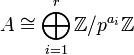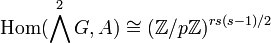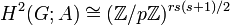# Formula for second cohomology group for trivial group action for abelian groups of prime power order

## Statement

### General case

Suppose$p$ is a prime number and$G$ and$A$ are finite abelian p-groups. Denote by$H^2(G;A)$ the second cohomology group for trivial group action of$G$ on$A$. Then,$H^2(G;A)$ is also a finite p-group and is given as follows:

Suppose:$\! A \cong \bigoplus_{i=1}^r \mathbb{Z}/p^{a_i}\mathbb{Z}$

and$\! G \cong \bigoplus_{j=1}^s \mathbb{Z}/p^{b_j}\mathbb{Z}$

Then:$\! H^2(G;A) \cong \operatorname{Ext}^1(G;A) \oplus \operatorname{Hom}(\bigwedge^2G,A)$

where:$\! \operatorname{Ext}^1(G;A) \cong \bigoplus_{1 \le i \le r, 1 \le j \le s} \mathbb{Z}/p^{\min\{a_i,b_j\}}\mathbb{Z}$

and$\! \operatorname{Hom}(\bigwedge^2G,A)\cong \bigoplus_{1 \le i \le r, 1 \le j < k \le s} \mathbb{Z}/p^{\min\{a_i,b_j,b_k\}}\mathbb{Z}$

Combining, we get that:$\! H^2(G;A) \cong \bigoplus_{1 \le i \le r, 1 \le j \le s} \mathbb{Z}/p^{\min\{a_i,b_j\}}\mathbb{Z} \oplus \bigoplus_{1 \le i \le r, 1 \le j < k \le s} \mathbb{Z}/p^{\min\{a_i,b_j,b_k\}}\mathbb{Z}$

Thus, we get the following formula for the prime-base logarithm of order:$\! \log_p|H^2(G;A)| = \sum_{1 \le i \le r, 1 \le j \le s} \min\{a_i,b_j\} + \sum_{1 \le i \le r, 1 \le j < k \le s} \min\{a_i,b_j,b_k\}$

### Case where the base group is elementary abelian

In the special case that$A$ is an elementary abelian group of prime power order, we get$A \cong (\mathbb{Z}/p\mathbb{Z})^r$ and hence:$\! \operatorname{Ext}^1(G;A) \cong (\mathbb{Z}/p\mathbb{Z})^{rs}$

and$\! \operatorname{Hom}(\bigwedge^2G,A)\cong (\mathbb{Z}/p\mathbb{Z})^{rs(s-1)/2}$

So that overall:$\! H^2(G;A) \cong (\mathbb{Z}/p\mathbb{Z})^{rs(s+1)/2}$

and thus:$\! \log_p|H^2(G;A)| = \frac{rs(s+1)}{2}$

## Facts used

1. Formula for second cohomology group for trivial group action in terms of Schur multiplier and abelianization